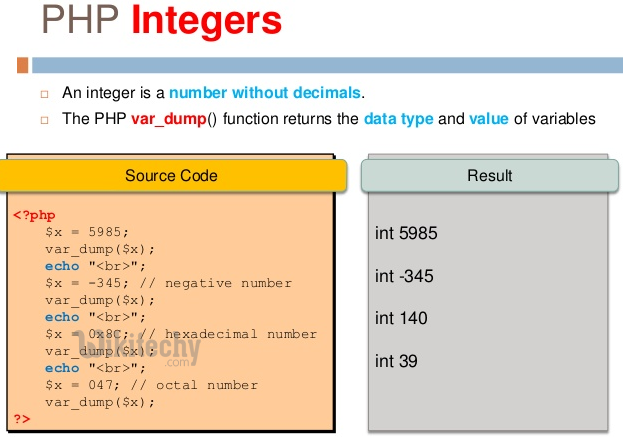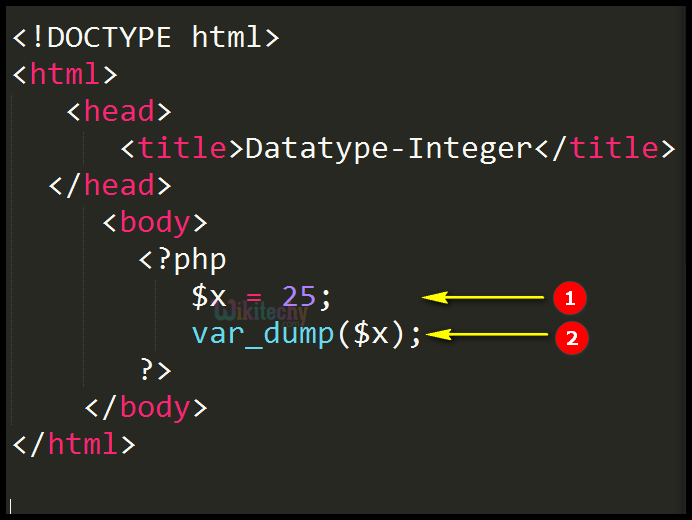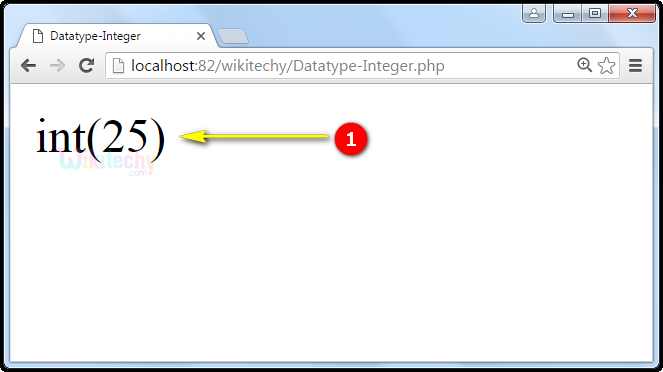# php tutorial - PHP Datatypes Integer - php programming - learn php - php code - php script

• Integer means numeric data types, which is also defined as a whole number with no fractional and decimal components.
• Integer may be lesser than or greater than or sometimes equals to zero.
• Integers can be specified in decimal (10-based), hexadecimal (16-based) or octal (8-based) notation, optionally preceded by a sign (- or +).## Syntax Explanation :

• Here \$x is an integer.
• \$x is an integer. The PHP var_dump() function returns the data type as integer with its integer value.

## php for beginners Code Explanation :1. In PHP, \$x = 25 is an integer variable that is holding the integer value =25.
2. The var_dump() function returns the integer data type with its integer value as 25.

## php tutorials Sample Output :1. In this output, var_dump() function returns the integer data type with its integer value as 25 i.e., int(25).